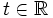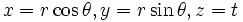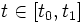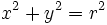# Right circular cylinder

## Definition

A right circular cylinder, or more precisely, a right circular cylindrical surface, is a union of all circles centered at points on a given line, of fixed radius, and each circle in the plane perpendicular to the line at that point. The line is termed the axis of the cylinder.

The above is the infinite right circular cylindrical surface. The finite version is obtained by replacing the line with an open line segment.

The term solid cylinder is used to refer to the unino of discs, instead of circles. The cylindrical surface is then termed the lateral surface of the solid cylinder.

### Terminology

• The line comprising centers is termed the axis of the cylinder.
• Planes perpendicular to the axis are termed cross-sectional planes
• The equal radius of all circles is termed the radius or base radius of the cylinder and is denoted by$r$
• For a finite cylinder, the length of the open line segment is termed the height of the cylinder and is denoted by$h$

## Equational descriptions

### Cartesian parametric description

If the axis is the$z$-axis, the parametric description is in terms of$t \in \R$ and$\theta$ an angle.$x = r \cos \theta, y = r \sin \theta, z = t$

For a finite cylinder, we have, instead of$t \in \R$,$t \in [t_0,t_1]$ where$t_1 - t_0 = h$.

### Cartesian equational description

If the axis is the$z$-axis, the equational description in Cartesian coordinates is:$x^2 + y^2 = r^2$

In other words, the$z$-coordinate is arbitrary, and any section with the$xy$-plane looks like a circle of radius$r$ centered at the origin.

## Structure and symmetry

Template:Direct product The infinite right circular cylinder is topologically, differentially, and geometrically the direct product of the circle$S^1$ and the real line$\R$. Moreover, this is an isometry-invariant factorization, in the sense that any isometry must take cross-sectional circles to cross-sectional circles, and lines parallel to the axis, to lines parallel to the axis.

Using this, we can compute that the group of isometries of the right circular cylinder is the semidirect product of the group itself (acting via the regular representation) and the Klein-four group, comprising the following four operations:

• The identity element
• Reflections about the$xy$-plane
• Rotation by an angle of$\pi$ about the$z$-axis
• Composite of the above two

Note that each of these isometries can be uniquely extended to isometrise fo$\R^3$, and that group of isometries is precisely the group of isometries of$\R^3$ that send the axis to itself as a line.

## Curvatures

The two principal directions at any point are the direction along the real line and along the circle. Along the real line, the curvature is 0, while along the circle, the curvature is the usual curvature associated with a circle. Thus, the Gaussian curvature at every point is zero.

## Constructions

### As a surface of revolution

This is the surface of revolution of: real line

The right circular cylinder can be obtained as the surface of revolution of a real line parallel to the axis of revolution. The perpendicular distance between the two lines in the radius of the cylinder.

### As a tube of a curve

This surface is the tube of the following curve: real line

The right circular cylinder can also be described as the tube of the curve which is the real line, and the radius of the tube is the same as the radius of the cylinder.

## Properties

Since there is an underlying group structure compatible with the metric, there is an isometry taking any point to any other point. Thus, the right circular cylider is homogeneous.

This is on account of the Gaussian curvature (the two-dimensional particular case of sectional curvature) being zero at every point.

## Related theorems

### Splitting theorem

This provides an instance of application of the following theorem: Splitting theorem

The splitting theorem states that any Riemannian manifold with everywhere nonnegative Ricci curvature can be expressed isometrically as a direct product of a line-free Riemannian manifold, and a Euclidean space.

In the case of the right circular cylinder, the Ricci curvature is everywhere nonnegative (in fact, everywhere zero) and the manifold can indeed be expressed as a direct product of the circle (which is line-free) and the real line (which is one-dimensional Euclidean space).

### Minding's theorem

This provides an instance of application of the following theorem: Minding's theorem

Minding's theorem states that two smooth surfaces with the same constant Gaussian curvature everywhere, must be locally isometric. Indeed, the cylinder and the plane both have everwhere zero Gaussian curvature, and the cylinder is locally isometric to the plane. This follows from the fact that the circle is locally isometric to a real line.